# Knowledge Base

## Initial wiggles in a very small transient stability case

A common question when a user first starts playing with very small transient stability test cases is “Why do I see wiggles in my plots with no disturbance?”  On large transient stability simulations there can be many different reasons for this, but for a 9 bus, 1 generator case you would rightly not expect to see any of this.  When PowerWorld staff see these support questions, very frequently it is related to the power flow convergence tolerance. You might see something like the following voltage profile at a bus.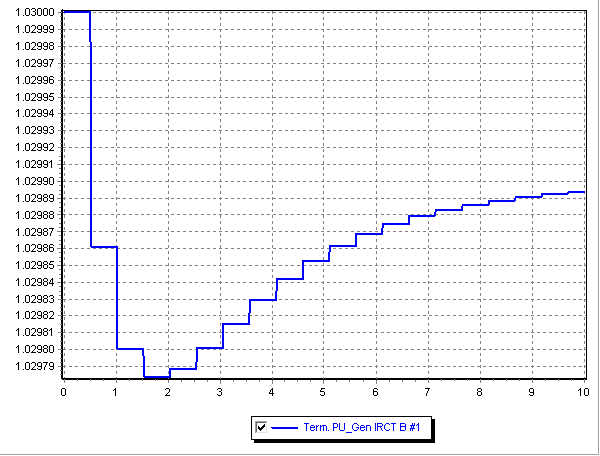First, realize this is basically a flat curve.  The variation is in the 5th significant digit from 1.0300 to 1.0298.  However, it is still moving.   If you see this kind of wiggle on a small case, the first culprit to check is the power flow convergence tolerance and the transient stability network boundary equation tolerance.  The power flow convergence tolerance is found on the Simulator Options under the Options\Common Options as shown in the following figure.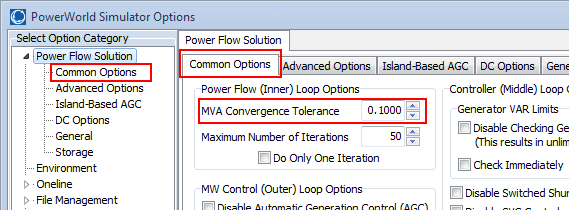Another location to look which wasn’t an issue in this particular customer’s example is the Transient Stability dialog’s Options\Power System Model\Network Equations Solution MVA Tolerance as shown in the following figure.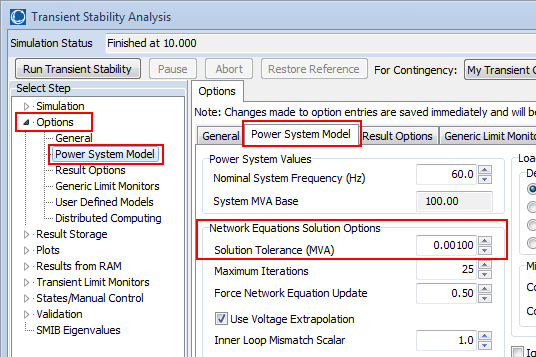In the example above the power flow convergence tolerance is 0.1 MVA which was a problem for this particular customer’s setup.  This case’s generation profile looks as follows.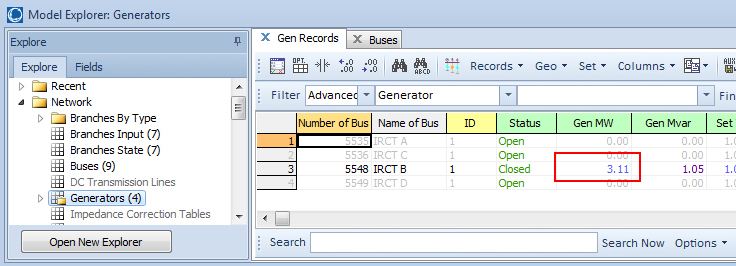Notice that there is only 3.11 MW of generation in this entire little case.  Now, we look at the bus mismatch table as follows in the Model Explorer.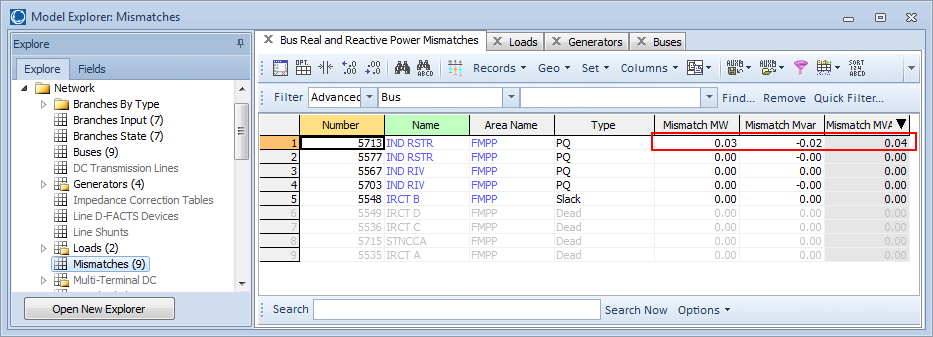Notice that it starts with a 0.03 MW mismatch.  This is below the MVA Convergence tolerance, but this means that the MW mismatch is in the 3rd significant digit of the total generation in the case.  The power flow solution serves as the initial condition for the transient stability run, so the power flow solution is an input to the stability run.  Thus it’s not too surprising than an input error in the 3rd significant digit results in a wiggle in the 5th significant digit of the output.

Thus, in this example, if we just change the power flow solution’s MVA convergence tolerance to 0.001 MVA, and resolve the power flow, we’ll end up with a more expected output as follows.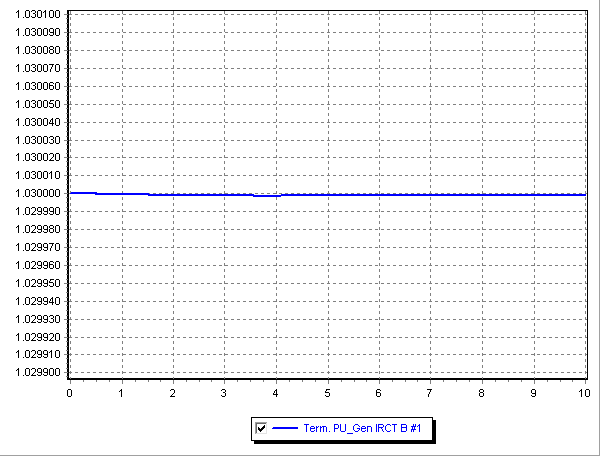June 12, 2013